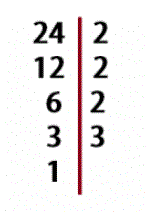Smartick is a fun way to learn math!Sep02

# What is Factorial Decomposition?

In today’s post we are going to answer the question, what is factorial decomposition? I hope that by the end of the post you’ll have it crystal clear.

#### Definition of Factorial Decomposition

We are going to start by remembering what a ”factor” is in mathematics. A factor is every number that is multiplied by another.

According to the definition, we can say that breaking down something into factors is transforming a number into the multiplication of other numbers.

For example, following this description, we could say that 30 could be broken down into 6 x 5.

Remember: To break down a number is to write its factors as the multiplication of other numbers.

#### Usefulness

But… what’s the point of breaking down a number into factors?

It can be used for many things, like helping us solve arithmetic equations.

For example: 15 x 8

We break down the 15 and 8 into factors.

15 x 8 = 3 x 5 x 2 x 4

Now we group the factors in a way that makes multiplication easier for us.

(2 x 5) x (3 x 4) = 10 x 12 = 120

But above all, the factorial decomposition of a number is very useful when we want to calculate the G.C.F (greatest common factor) or the L.C.M (least common multiple). To facilitate this task we need factorial decomposition to be done in prime factors, therefore all the factors that the number is made up of are only prime numbers.

If you would like to review how to calculate G.C.F and L.C.M, check out these posts:

#### Calculate

In order to calculate all of the prime factors of a number, you have to go about dividing the original number by its factors until reaching 1.

For example, breaking down the factorial 24 is done like this:You can see how to do it step by step in this post on our blog: Follow 8 Easy Steps to Factor a Number.

I suppose that after this review and reading the previous recommended posts you will know how to answer the question, What is factorial decomposition?